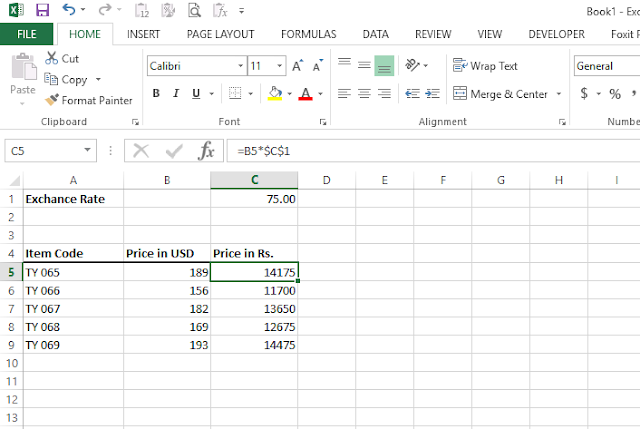# The Currency Converter in excel

## The Currency Converter in excel

### Currency Calculator in excel for Exchange

In this Microsoft Excel blog post, of The Currency Converter, we'll tell you how you can find the currency exchange rate of any currency and convert it to another currency, with the help of an easy-to-use Excel formula, as you know. The currency of any country cannot be operated in any other country due to which you have to exchange that currency and the currency exchange rate of each country is different.

So let's get started.
1. The first thing you need to do is write down the currency exchange rate in column C.
2. Then write the item code in column A as you can see in the picture below.
3. And now in column B, I have to write this currency as I have written for example USD.
4. Now put the final formula in column C which will let you know if the rate of this item is so much in USD then how much in other currency.

You will have the answers to these questions after this formula.

How to currency converter in excel?
Currency converter for excel?
The Currency converter on excel?
Currency converter in excel?
Currency converter formula?
Currency converter formula excel?
Currency converter excel?